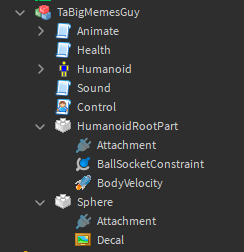# Help with sphere controls (Complex issue)

Hi, I was scripting the controls for a sphere character and I’ve ran into an issue. The controls only work as intented when you look one axis relative to `HumanoidRootPart`.

If you face your camera opposite of the the sphere, the controls are reversed and forwards is now backwards, left is now right, e.t.c…

This is because i update the body mover, `BodyVelocity` of the `HumanoidRootPart` using `X, Y, Z` which is relative to the cube.

My intended behaviour is that the controls are relative to where the camera is facing (Forward will always go towards where you’re looking, no matter where you look.)

My attempts are the following:

• Modify the `CFrame` of the `HumanoidRootPart` relative to the camera (Didn’t work).

• Modify the `Orientation` property of the `HumanoidRootPart`, so that it face the way the camera is facing (Moved a bit then moved back to it’s original orientation).

• Modify the `BallSocketConstraint`'s properties to see if it helps (Didn’t work).

My last option was to ask on here so any help is appreciated.

Information:

Client Control Script
``````-- Services --
local ContextActionService = game:GetService("ContextActionService")
local UserInputService = game:GetService("UserInputService")
local RunService = game:GetService("RunService")
local Workspace = game:GetService("Workspace")

-- Variables --
local Character = script.Parent
local Humanoid = Character.Humanoid
local HumanoidRootPart = Character.HumanoidRootPart
local BodyVelocity = HumanoidRootPart.BodyVelocity
local Camera = Workspace.CurrentCamera

local WalkSpeed = Humanoid.WalkSpeed
local JumpPower = Humanoid.JumpPower
local Force = 50

local ForwardApplied = 0
local BackwardsApplied = 0
local RightApplied = 0
local LeftApplied = 0

local KeyMap = {
Forwards = {Enum.KeyCode.W};
Backwards = {Enum.KeyCode.S};
Right = {Enum.KeyCode.D};
Left = {Enum.KeyCode.A};
}

-- Functions --
local function ForwardInput(Action, State, Object)
if Action == "Forwards" then
if State == Enum.UserInputState.Begin then
ForwardApplied = -(WalkSpeed * Force)
BodyVelocity.Velocity += Vector3.new(0, 0, ForwardApplied)
elseif State == Enum.UserInputState.End then
BodyVelocity.Velocity -= Vector3.new(0, 0, ForwardApplied)
end
end
end

local function BackwardsInput(Action, State, Object)
if Action == "Backwards" then
if State == Enum.UserInputState.Begin then
BackwardsApplied = (WalkSpeed * Force)
BodyVelocity.Velocity += Vector3.new(0, 0, BackwardsApplied)
elseif State == Enum.UserInputState.End then
BodyVelocity.Velocity -= Vector3.new(0, 0, BackwardsApplied)
end
end
end

local function RightInput(Action, State, Object)
if Action == "Right" then
if State == Enum.UserInputState.Begin then
RightApplied = (WalkSpeed * Force)
BodyVelocity.Velocity += Vector3.new(RightApplied, 0, 0)
elseif State == Enum.UserInputState.End then
BodyVelocity.Velocity -= Vector3.new(RightApplied, 0, 0)
end
end
end

local function LeftInput(Action, State, Object)
if Action == "Left" then
if State == Enum.UserInputState.Begin then
LeftApplied = -(WalkSpeed * Force)
BodyVelocity.Velocity += Vector3.new(LeftApplied, 0, 0)
elseif State == Enum.UserInputState.End then
BodyVelocity.Velocity -= Vector3.new(LeftApplied, 0, 0)
end
end
end

-- Events --
RunService.RenderStepped:Connect(function(Delta)
--TODO: Update HumanoidRootPart so that it faces the same way the camera is.
end)

Humanoid.Changed:Connect(function(Property)
if Property == "WalkSpeed" then
WalkSpeed = Humanoid.WalkSpeed
elseif Property == "JumpPower" then
JumpPower = Humanoid.JumpPower
end
end)

-- Binds --
ContextActionService:BindActionAtPriority("Forwards", ForwardInput, false, 2000, unpack(KeyMap.Forwards))
ContextActionService:BindActionAtPriority("Backwards", BackwardsInput, false, 2000, unpack(KeyMap.Backwards))
ContextActionService:BindActionAtPriority("Right", RightInput, false, 2000, unpack(KeyMap.Right))
ContextActionService:BindActionAtPriority("Left", LeftInput, false, 2000, unpack(KeyMap.Left))
``````
ExplorerVideos:

Intended behaviour

Unintended behaviour

(Notice how the controls are inverted when I look behind me)

I know what the issue is, I’m just unsure how to fix it.

1 Like

Have you tried to use Camera’s normals to move the sphere relative to the camera’s rotation?

Example with Forward and Left:

``````local Camera = workspace.CurrentCamera

local function ForwardInput(Action, State, Object)
if Action == "Forwards" then
if State == Enum.UserInputState.Begin then
ForwardApplied = -(WalkSpeed * Force)
BodyVelocity.Velocity += (Camera.CFrame.LookVector * ForwardApplied)
elseif State == Enum.UserInputState.End then
BodyVelocity.Velocity -= (Camera.CFrame.LookVector * ForwardApplied)
end
end
end

local function LeftInput(Action, State, Object)
if Action == "Left" then
if State == Enum.UserInputState.Begin then
LeftApplied = -(WalkSpeed * Force)
BodyVelocity.Velocity += (-Camera.CFrame.RightVector * LeftApplied)
elseif State == Enum.UserInputState.End then
BodyVelocity.Velocity -= (-Camera.CFrame.RightVector * LeftApplied)
end
end
end
``````
2 Likes

This doesn’t work unfortunately because the number is applied to a `BodyVelocity`.

1 Like